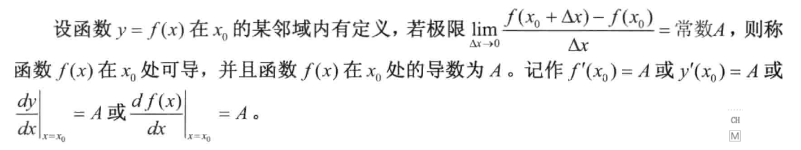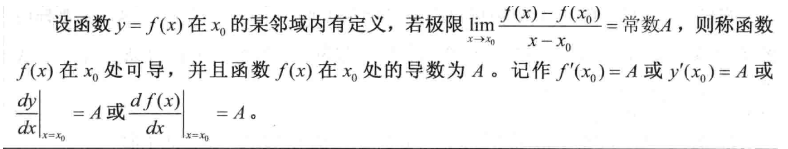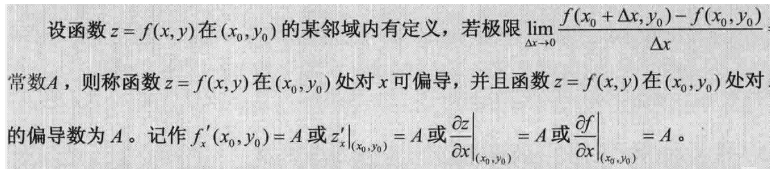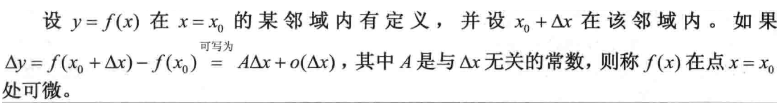• 一元函数，多元函数，可微的含义：就是用极限的思想近似反应两个变因素之间的函数关系。近似代替。 一元函数微分的几何意义：就是曲线x增加了一部分，y增加多少的表示。用了极限分割的思想，无线接近。dy与△y...
目录

一元函数，多元函数，可微的含义

多元函数微分的几何意义
多元函数偏导
展开全文• 基础知识: 一元函数的连续性: 前提: 1 函数f(x)在点x。有定义 2 (是个常数) 3 相等,即 二元函数的连续条件: 在某点导: 定义1: 定义二: 偏导: 可微: ...
基础知识:

lim

⁡

x

→

x

0

−

f

(

x

)

=

lim

⁡

x

→

x

0

+

f

(

x

)

=

A

⇔

lim

⁡

x

→

x

0

f

(

x

)

=

A

\lim _{x \rightarrow x_{0}^{-}} f(x)=\lim _{x \rightarrow x_{0}^{+}} f(x)=A \Leftrightarrow \lim _{x \rightarrow x_{0}} f(x)=A

lim

⁡

x

→

x

0

−

f

(

x

)

≠

lim

⁡

x

→

x

0

+

f

(

x

)

⇒

lim

⁡

x

→

x

0

f

(

x

)

\lim _{x \rightarrow x_{0}^{-}} f(x) \neq \lim _{x \rightarrow x_{0}^{+}} f(x) \Rightarrow \lim _{x \rightarrow x_{0}} f(x)

不存在但不是

∞

\infty

一元函数的连续性:
前提:
1 函数f(x)在点x。有定义2

l

i

m

x

→

x

0

−

f

(

x

)

lim _{x \rightarrow x_{0}^{-}} f(x)

必须存在 (是个常数)3 相等,即

lim

⁡

x

→

x

0

f

(

x

)

=

f

(

x

0

)

\lim _{x \rightarrow x_{0}} f(x)=f\left(x_{0}\right)

二元函数的连续条件:

lim

⁡

(

x

,

y

)

→

(

x

0

,

y

0

)

f

(

x

,

y

)

=

f

(

x

0

,

y

0

)

\lim _{(x, y) \rightarrow\left(x_{0}, y_{0}\right)} f(x, y)=f\left(x_{0}, y_{0}\right)

在某点可导:
定义1:定义二:可偏导:可微:展开全文• 在高等数学一元函数微分学中研究的关键问题之一是导和可，夹杂着函数连续，简短等知识点，这几个相关的概念混在一块总是难以理解，什么导一定可微导一定连续之类的。 这里把这几个概念就自己的理解做一下...
在高等数学一元函数微分学中研究的关键问题之一是可导和可微，夹杂着函数连续，简短等知识点，这几个相关的概念混在一块总是难以理解，什么可导一定可微，可导一定连续之类的。 这里把这几个概念就自己的理解做一下解释。 1.极限。 求某一数列趋近于无穷的情况，某一函数趋近于无穷的情况，某一函数趋近于某一点的情况。只与自变量（数列为项数）和表达式有关。 2.连续，可导，可微。 连续，可导，可微三个均涉及到自变量，因变量，表达式，定义上又略有不同。
(这里个很重要的概念：增量。即Δx，Δy。很多人不知道增量的意思和dx，dy代表什么意思，其实读书的时候仔细一点，这些概念都有提到。)

2.1 连续 2.1.1 定义1：当函数自变量的增量无线趋近于0的时候，因变量的增量的极限等于0。 定义2：当自变量趋近于X0的时候，函数极限等于函数值f(x0). 2.1.2 理解 连续也只涉及到自变量向趋近于x0时，y的极限。只不过极限算完就算完了，极限是否存在，存在的话值是多少都不是我们关心的问题，但在连续的定义里面。要根据极限的计算结果对函数连续性进行评价。函数在极限存在且在该点有定义。极限不存在怎么办？该点没定义怎么办？于是就像"缺什么补什么"似的，高数引进的间断点的概念，并且根据函数该点处的极限情况分成了第一类间断点和第二类间断点。 请注意，关于连续，我们经常研究的是某一点的情况。
	对于很多非数学系的工科同志而言，这里又有一个点常常被忽略——有定义（包含分母不为0这种没定义的情况）

2.2 可导与导数 2.2.1 定义 当自变量趋近于某一点x0的时候，因变量-f(x0)/自变量-x0的极限存在。 2.2.2 理解 如果说连续的定义和计算与极限还有几分相似的话，可导和可微就完全不是了。可导相当于构造了一个新的函数g(x),计算该函数的极限。当然，新构造出来的函数有它自己的实际意义，也就是因变量增量的比与自变量增量的比的极限。 另一个值得注意的就是可导的结果并不是一个实际的值，好像布尔值一样，对函数某点做是否可导判断的问题可转换为函数g(x)在该点的极限是否存在。得出是或否就行了。 到这里，理解“可导一定连续，但连续不一定可导”应该不难了，因为你肯定在极限中做过让你头疼很久的0比0型极限，通常情况是一个极限单独算存在，合在一块经过有限加减运算刚好凑成了0/0型，如果连续的结果（某一点的极限存在）存在，把它看成一个有结果的极限，那么可导刚好类似于0/0型。所以只知道连续的结果算不出可导的结果。知道了可导的结果，就知道连续的极限存在，也知道了可导一定连续。 如果感觉记不住，就用一种地球人都知道的记忆方法：你看到面前的一排小蓝车，你把第一辆车推到了，接着后面的车跟着也倒了，可倒（导）一定连续，连续不一定可倒（导）。
	请注意：对于导数是否存在一般为某一点，导数则有可能是一个区间

2.3 可微
	注意dx和dy从现在起才正式出现。

2.3.1 定义 Δy可以写成线性的形式A*Δx加上一个无穷小，即线性的形式。A与x无关，无穷小相对于Δx而言。满足条件的成为函数可微，并称AΔx为x在x0处的微分。记为dy，自变量的增量Δx等于自身的微分dx，所以有dy=Adx。 2.3.2 解释 2.3.2.1 Δy与dy的区别 Δy与dy之间相差了一个无穷小量。也可以说dy是Δy的近似。 2.3.2.2 可微和可导一样，判断是否可微即可，但A的确定又是一个难题，对于学高等数学的工科生直接使用结论A即为该点的导数值即可。
	请注意：可微相对于某一点来说，微分相对于定义域

展开全文• （一）一元函数微分学基础 这一部分只会讨论什么是导数与微分，以及它们的计算。也是一元函数微分学最基础的部分。 1）讨论导数与微分的概念 给出函数判断导数是否存在： 利用导数的定义判断在某一点导数是否存在，...


文章目录
前言(一)一元函数微分学基础1）讨论导数与微分的概念2）导数与微分的计算
(二)导数的应用1）通过导数定义的属性2）通过导数计算的属性3）与导数间接相关的属性4）函数的应用题（多为最值问题）
(三)中值定理1）不等式问题a. 具体函数不等式问题b. 抽象函数不等式问题
2）零点问题a. 可导具体函数的零点问题b. 证明存在

f

(

ξ

)

=

0

f(ξ)=0

的零点问题c. 证明存在

f

′

(

ξ

）

=

0

f^{'}(ξ）=0

的零点问题d. 双中值问题e. 复合函数

ψ

(

x

,

f

(

x

)

,

f

′

(

x

)

)

ψ(x,f(x),f^{'}(x))

的零点f. 复合函数

ψ

(

x

,

f

(

x

)

,

f

′

(

x

)

,

f

′

′

(

x

)

)

ψ(x,f(x),f^{'}(x),f^{''}(x))

的零点g. 存在某ξ满足某不等式3) 多说一句

前言
本笔记不涉及基础知识，重点在于分析考研数学的出题角度和对应策略。笔记随着做题的增多，不定时更新。且为了提高效率，用表线性梳理的形式代替思维导图，望谅解。
如有缺漏错误，欢迎补充指正！
这一章的特点是出题点较多且杂，其实考察的知识就是大纲上的那些。或者说出题的角度灵活比较合适。 除了掌握大纲中的要求，还要多做练习题找到题中经常出现的坑，大都是对定义的精确考察，我把遇到的都记录在这里。
(一)一元函数微分学基础
这一部分只会讨论什么是导数与微分，以及它们的计算。也是一元函数微分学最基础的部分。
1）讨论导数与微分的概念
给出函数判断导数是否存在：
利用导数的定义判断在某一点导数是否存在，注意可导必定连续。如果函数是分段函数，要保证左右导数都存在且相等才存在导数。如果函数是绝对值函数，也要讨论左右导数是否相等，有时需要利用连续或极限的保号性。另外绝对值函数导数存在的常用条件为绝对值函数的导数为0.如果是由极限式表示的函数，应先求出此极限，得到

f

(

x

)

f(x)

的分段表达式，再讨论

f

(

x

)

f(x)

的可导性。
给出函数及其性质判断其它函数的可导性：
设有函数

f

(

x

)

f(x)

在

x

=

0

x=0

处存在二阶导数，那么在

x

=

0

x=0

的某邻域内，当

x

≠

0

x\not=0

时，

f

′

(

x

)

f^{'}(x)

存在, 但

f

′

′

(

x

)

f^{''}(x)

不一定存在。函数的和式存在导数，不能证明分式的导数都存在，对于导数的定义也是如此。
给出函数及性质求待定参数
利用导数定义及题中条件即可。
2）导数与微分的计算
可能出现的导数形式：
基本初等函数的导数及其复合（公式记牢）变限积分（求导公式、变量代换）隐函数求导（直接求导）反函数求导（

d

x

d

y

=

1

d

y

d

x

\frac{dx}{dy} = \frac{1}{\frac{dy}{dx}}

,注意对谁求导，链式法则）参数式函数求导（链式法则）求解高阶导数（链式法则）求函数的n阶导数（泰勒公式、常用n阶导数公式、级数 ）
(二)导数的应用
当充分理解什么是导数后，我们重新回到函数部分，思考导数在函数的计算和性质中可以有什么应用。 所以标题为导数的应用，也可以称为函数的性质。
导数在大纲中有以下应用： 极值、最值、单调性、凹凸性、拐点、驻点、渐进线、曲率、曲率半径、曲率圆、画出函数草图。
其中，拐点与驻点是通过导数定义的属性。
极值、单调性、凹凸性、曲率、曲率半径、曲率圆是本来有自己的定义，但通常需要用导数来计算和确定的属性。
最值和渐近线是间接和导数有关系的属性。
1）通过导数定义的属性
驻点：连续曲线

y

=

f

(

x

)

y = f(x)

上

f

′

(

x

)

=

0

f^{'}(x) = 0

的点。与驻点附近

f

′

(

x

)

f^{'}(x)

的值没有关系。拐点：连续曲线

y

=

f

(

x

)

y=f(x)

上凹、凸弧的分界点。注意：

f

′

′

(

x

)

=

0

f^{''}(x) = 0

, 左右邻域内

f

′

′

(

x

)

f^{''}(x)

异号只是拐点的充分条件。如果在

x

=

x

0

x=x_0

处函数有定义，左右邻域内

f

′

′

(

x

)

f^{''}(x)

异号,但

f

′

′

(

x

0

)

f^{''}(x_0)

不存在，

x

=

x

0

x = x_0

仍为驻点。
2）通过导数计算的属性
单调性：判断函数单调性，首先便是导数。之后通过讨论或者变形判断区间上的导数的正负性。极值：最准确确定极值的方法是通过极值的定义。导数是提供了判断可导点处极值的必要条件(

f

′

(

x

)

=

0

f^{'}(x) = 0

)和两个充分条件(①

f

′

(

x

)

=

0

f^{'}(x) = 0

, 左右邻域内

f

′

(

x

)

f^{'}(x)

异号 ②

f

′

(

x

)

=

0

f^{'}(x) = 0

,

f

′

′

(

x

)

=

a

(

a

≠

0

)

f^{''}(x) = a(a \not= 0)

)凹凸性：最准确确定凹凸性的方法是通过凹凸性的定义。导数是提供了判断可导点处凹凸性的必要条件(

f

′

′

(

x

)

=

0

f^{''}(x) = 0

)和两个充分条件(①

f

′

′

(

x

)

=

0

f^{''}(x) = 0

, 左右邻域内

f

′

′

(

x

)

f^{''}(x)

异号 ②

f

′

′

(

x

)

=

0

f^{''}(x) = 0

,

f

′

′

′

(

x

)

=

a

(

a

≠

0

)

f^{'''}(x) = a(a \not= 0)

)曲率：曲率部分只需要关注三个公式。曲率公式：

k

=

∣

y

′

′

∣

(

1

+

y

′

2

)

3

2

k = \frac{|y^{''}|}{(1+y^{'2})^{\frac{3}{2}}}

, 曲率半径：

R

=

1

k

R = \frac{1}{k}

，曲线上点

M

(

x

,

y

)

M(x,y)

处的曲率中心的坐标

(

α

，

β

)

(α，β)

为

α

=

x

−

y

′

(

1

+

y

′

2

)

y

′

′

α=x-\frac{y^{'}(1+y^{'2})}{y^{''}}

，

β

=

y

+

1

+

y

′

2

y

′

′

β=y+\frac{1+y^{'2}}{y^{''}}

3）与导数间接相关的属性
最值：从所有驻点、不可导点、闭区间两端点中寻找最大值与最小值。渐进线：从

lim

⁡

x

→

x

0

\lim\limits_{x\to x_{0}}

=

∞

=\infty

判断铅直渐近线，从

lim

⁡

x

→

+

∞

=

a

\lim\limits_{x\to+\infty} =a

或

lim

⁡

x

→

−

∞

=

a

\lim\limits_{x\to-\infty} =a

判断水平渐近线，通过

lim

⁡

x

→

+

∞

f

(

x

)

x

=

a

\lim\limits_{x\to+\infty}\frac{f(x)}{x} = a

和

lim

⁡

x

→

+

∞

(

f

(

x

)

−

a

x

)

=

b

\lim\limits_{x\to+\infty}(f(x)-ax)=b

来判断斜渐近线

y

=

a

x

+

b

y=ax+b

是否存在。
4）函数的应用题（多为最值问题）
若实际问题必定有最值，且由问题建立的表达式只有一个驻点，那么该驻点便是极值点。该类应用题常于解析几何或位置参数的函数联系，需要建立目标函数或讨论参数。
(三)中值定理
这一部分是一元函数微分学的难点。
导数是刻画函数在一点处变化里的概念，它反映的是函数在一点邻近的局部变化性态。
但在理论研究和实际应用中，常常需要知道函数在某一区间上的整体变化情况和它在区间内某些点处的局部变化性态之间的关系。
有关中值定理的题型解题方法可能是不唯一的，可以用拉格朗日中值解，说不定也可以用罗尔定理解。
关于函数某一区间变化情况或某些点处的局部变化性态问题求解方式有以下几种：
利用导数讨论单调性最值存在极值定理结合费马定理介值定理积分中值定理罗尔定理拉格朗日中值定理拉格朗日余项泰勒公式柯西中值定理
以及几种解题技巧：
积分法微分方程法函数与导数存在零点个数的关系
下面我们根据问题的提问方式具体分析，中值定理的题型大概可分为以下几类：
1）不等式问题
这类问题放在第一类，是因为不等式问题求解方式众多。比如我们以前提到的利用条件极值解不等式问题，在这一章还可以利用导数用单调性解不等式问题，以及各种中值定理和泰勒公式求解。
a. 具体函数不等式问题
不管具体不等式有没有明显利用中值定理的特征，首先考虑使用中值定理，简单尝试后若发现利用条件不足以证明，换用导数求解。在利用导数讨论单调性求解时，最坏的情况便是拆分多个区间具体讨论和层层求导，这时需要有耐心，考试的解题主体在这里的情况应该会很少发生，但也不能排除。纯导数的题真的会考的，2011年数学一考过一次。
b. 抽象函数不等式问题
抽象函数不等式问题不考虑使用导数证明，若题目中出现

f

′

′

(

x

)

f^{''}(x)

的条件或需要证明的不等式中出现了

f

′

′

(

x

)

f^{''}(x)

或者更高阶导数，考虑使用拉格朗日余项泰勒公式证明。若题目中仅出现

f

(

x

)

f(x)

和

f

′

(

x

)

f^{'}(x)

，考虑使用罗尔定理、拉格朗日中值定理、柯西中值定理、极值最值证明。
2）零点问题
a. 可导具体函数的零点问题
一般构造函数，利用导数讨论单调性和边界点值、最值和极值、最后利用介值定理判断零点个数。带有参数的具体函数，需要对参数进行讨论。
b. 证明存在

f

(

ξ

)

=

0

f(ξ)=0

的零点问题
这类问题的特点是具体函数的导数过于复杂不容易判断以及抽象函数给出

f

(

x

)

f(x)

,求解

f

(

ξ

)

=

0

f(ξ)=0

的问题。因为题目中的条件和要求都和导数无关，若想利用中值定理，就要对函数求积分，对原函数

F

(

x

)

F(x)

利用中值定理求解零点。即“积分法”。
c. 证明存在

f

′

(

ξ

）

=

0

f^{'}(ξ）=0

的零点问题
这类问题一般解法是利用最值极值定理，好处是不管

f

′

(

x

)

f^{'}(x)

是否连续，只要通过条件判断出最值不在端点处，区间内必定存在极值点，在极值点处

f

′

(

ξ

)

=

0

f^{'}(ξ)=0

.相应的，这类问题的一大坑便是想当然的认为导数连续，即使函数连续且处处可导，其导函数也不一定连续。而导函数不连续，便不能使用介值定理。
d. 双中值问题
双中值意思就是证明要求中出现ξ、η两个不确定的量。求解方式和单中值大同小异，注意两个中值之间的关系就好。
e. 复合函数

ψ

(

x

,

f

(

x

)

,

f

′

(

x

)

)

ψ(x,f(x),f^{'}(x))

的零点
这类题型的特点是题目要求的证明涉及

x

,

f

(

x

)

x,f(x)

,和

f

′

(

x

)

f^{'}(x)

。比如根据题目条件证明：

(

1

+

ξ

)

f

′

(

ξ

)

−

f

(

ξ

)

=

0

(1+ξ)f^{'}(ξ)-f(ξ)=0

如果单纯用

f

(

x

)

f(x)

结合罗尔定理、拉格朗日中值定理或泰勒公式，一定是求不出的。因为要求中的ξ同时出现在x,f(x),f(x)的导数中。使用拉格朗日余项泰勒公式时有个坑，余项中的ξ并不是常数，会随着

x

x

和

x

0

x_0

的变化而变化，带入不同的值一定要区分

ξ

1

ξ_1

和

ξ

2

ξ_2

。这类题型用这种错误的不区分的方法虽然也能凑出来，但是错误的，千万不能用。这类题型的基本思路是“构造”。基本方法是微分方程法和柯西中值定理。微分方程法就是构造出一个函数，其满足罗尔定理，通过罗尔定理求解。因为新构造的函数中，f(x)只是整个函数的一部分，新构造的函数为复合函数，所以罗尔定理才有可能正好是所要求的证明。柯西中值定理本质上也是构造，需要构造一个新函数g(x)来满足柯西中值定理的条件。
f. 复合函数

ψ

(

x

,

f

(

x

)

,

f

′

(

x

)

,

f

′

′

(

x

)

)

ψ(x,f(x),f^{'}(x),f^{''}(x))

的零点
涉及到

f

′

′

(

x

)

f^{''}(x)

的时候，有两种处理方式，罗尔定理或拉格朗日中值定理使用两次或利用拉格朗日余项泰勒公式。对于函数f(x)，要证明存在

f

′

′

(

ξ

)

=

0

f^{''}(ξ)=0

，需要有至少三个零点。涉及到

f

′

′

′

(

x

)

f^{'''}(x)

的时候，大概只有拉格朗日余项泰勒公式。
g. 存在某ξ满足某不等式
证明形式比如：

f

′

(

ξ

)

<

a

f^{'}(ξ)< a

把存在某ξ放在零点问题中，是因为解题思路和零点问题非常相似。根据出现的函数或导数的阶数判断使用什么方法，最后判断一下ξ的范围，或者进行一下放缩来证明不等式。
3) 多说一句
涉及中值定理和不等式的问题灵活多样，列举这些不同的题型只是在做题的时候能更快找到思路。还有很多不在上述列举中的题目，这时就需要结合中值定理发挥想象力，求解出来。这也是为什么不等式和中值定理是难点和重点。
展开全文• 前言 本笔记不涉及基础知识，重点在于分析考研数学的出题角度和对应策略。笔记随着做题的增多，不定时...在还未接触多元微分以及积分时，我记得一元函数积分学的难度是高于极限的——要好好整理。 一元函数微分学 ...笔记
• 附上可微的定义： ...rank_v2~rank_v29-1-111131869.nonecase&utm_term=%E5%8F%AF%E5%BE%AE&spm=1018.2226.3001.4450 答案： 资料1： 资料2： 资料3：
• 二阶导点是拐点的必要条件 设存在，且点 （x0，f(x0) )为曲线拐点，则 判断拐点的第一充分条件 设 f(x) 在 x=x0 处连续，在点 x=x0 的某去心邻域内二阶导数存在，且在该点的左右邻域内变号 则点 （x0，f(x0)...
• 一阶导点是极值点的必要条件 设 f(x) 在 x=x0 处导，且在点 x0 处取得极值，则必有 判断极值的第一充分条件 设 f(x) 在 x=x0 处连续，且在 x0 的某去心邻域内导 x0 极小值点 ...
• 一元函数微分学 主要涉及两方面: 概念类。主要是定义，通过一个式子来判断它的导数(存不存在、是多少)。 计算类。(包括很多 分段函数 复合函数 反函数 参数方程 隐函数 对数求导 幂指函数 高阶导数 变限积分求导) ...
• 函数 $f:\bbR^n\to \bbR$ 在 $\bbR^n\bs \sed{0}$ 可微, 在 $0$ 连续, 且 $$\bex\lim_{\bbx\to0}\frac{\p f(\bbx)}{\p x_i}=0,\ i=1,2,\cdots,n. \eex$$ 证明 $f$ 在 $0$ 可微. 证明: 由 \beex \bea |f(\bbx)-f...
• 一元函数微分 1.导数定义 2.左右导数导数的几何意义和物理意义 3.函数的导性与连续性之间的关系 4.平面曲线的切线和法线 5.四则运算法则 6.基本导数与分表 7.复合函数，反函数，隐函数以及参数方程所确定的函数...
• 第二讲 一元函数微分学 一、导数定义 $f'(x_0) = \lim_{\Delta x \to 0} \frac{f(x_0 + \Delta x) - f(x_0)}{\Delta x}$ 导数存在的充要条件 $f'(x) \,\exists \iff f'_-(x_0) = f'_+(x_0)$ 左导数 \(f'_-(x_0...
• 在上一讲的末尾我们谈到，在实际的工程当中我们常常借助计算机程序，利用迭代法进行极值的求取，这里我们首先从一元函数入手，看看如何通过这种方法找到一元函数的极值点。 1.起步：用牛顿法解方程 1.1.原理分析 在...自然语言处理 自动驾驶 神经网络 tensorflow 深度学习
• ## 为什么偏导数连续，函数就可微？

万次阅读 多人点赞 2018-10-23 17:50:27
如果函数 的偏导数 、 在点 连续，那么函数在该点可微。 下面来解释这个结论，并且减弱这个结论的条件。 先简单阐述下“连续”、“偏导数”、“可微”的意义，后面要用到。如果非常熟悉了，可以直接跳到最后...偏导数 高等数学
• 文章目录前言理解一元函数微分理解二元函数微分与全微分总结 ...一元函数f(x)在x = a可微，即指f(x)在x = a点的切线g(x)距离实际值 f(a) 即x = a附近的实际值足够接近，以至于当x无限趋近于a时，可以用g(x)来拟合f(x)数学 数学竞赛 人工智能 机器学习 高等数学
• 定理 (1) 带拉格朗日余项的 n 阶泰勒公式 设 f(x) 在点 x0 的某个邻域内 n+1 阶导数存在，则对该邻域内的任意点 x ，有 (2) 带佩亚诺余项的 n 阶泰勒公式 ...重要函数的麦克劳林展开式 ...
• ## 函数可微的定义

千次阅读 2020-12-13 16:54:50
附：导数与微分https://wenku.baidu.com/view/676a08b065ce05087632131f.html
• 定理 设 f(x) 满足 [a,b]上连续 (a,b)内导 则 使得 或者写成
• 第二章 一元函数微分学1.导数与微分，导数的计算知识点微分的定义导数运算法则基本初等函数导数公式变限积分求导参数式、隐函数、幂指函数、反函数 求导解题思路2.导数的应用知识点相关定义凹凸性判断拐点的条件渐近...
• 文章目录第二章 一元函数的导数与微分概念及其计算一、一元函数的导数与微分二、按定义求导数及其适用的情形三、基本初等函数导数表，导数四则运算法则与复合函数微分法则（一）基本初等函数导数表（分表）四、...
• 1、原函数（不定积分）存在定理 （1）连续函数 f(x) 必有原函数 F(x) （2）含有第一类间断点、无穷间断点的函数 f(x) 在包含该间断点的区间内必没有原函数 F(x) ...另一个充要条件：若 f (x)在 [a,b] 上...
• 文章目录1、函数f(x)f(x)f(x)在点x0x_0x0​极限存在的充要条件2、函数f(x)f(x)f(x)在点x0x_0x0​连续的充要条件3、函数f(x)f(x)f(x)在点x0x_0x0​可微3.1一元函数可导的充要条件3.2多元函数偏导的定义4、函数f(x)f(x...数学 机器学习
• 连续，那么函数在该点可微。 下面来解释这个结论，并且减弱这个结论的条件。 先简单阐述下“连续”、“偏导数”、“可微”的意义，后面要用到。如果非常熟悉了，可以直接跳到最后一节“偏导数连续推出可微”。 1 ...经验分享
• 文章目录第三章 一元函数积分概念、计算及应用 第三章 一元函数积分概念、计算及应用
• 知识点 导数与微分，导数的计算 定义 导数。[《全书》P36] 左、右导数。[《全书》P37] 函数的微分。[《全书》P37] 函数的微分。...导与可微的关系。[《全书》P38] 函数的微分与函数的增量之间...
• 最近复习到高数的一元函数微分部分 ，导和可是两个特别重要也特别容易混淆的概念。所以简单记录一下，便于自己理解，仅供参考。 导数 从物理角度来说（牛顿是从物理学的角度发明出的积分）某点的导数就是一个...
• 大学生数学竞赛（非数学）证明一元函数有界性常用方法 什么是有界函数： 有界函数是设f(x)是区间E上的函数，若对于任意的x属于E，存在常数m、M，使得m≤f(x)≤M，则称f(x)是区间E上的有界函数。其中m称为f(x)在...
• 2）判断拐点的第二充分条件： 目标点处（邻域）函数三阶导； 目标点处二阶导为 0 ； 目标点处三阶导不为 0 。 3）判断拐点的第三充分条件： 目标点处 n 阶导； 前 n-1 阶导数为 0 ，n 阶导数不为 0 ； n 为奇数...思维导图 数学 高等数学...